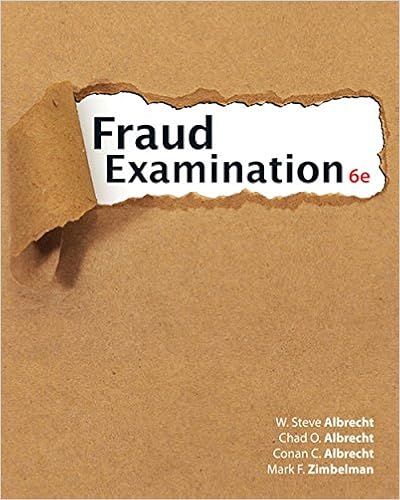# LIN_Practice_Final_Solutions.pdf - Johns Hopkins University...

• Test Prep
• 13

This preview shows page 1 - 4 out of 13 pages.

##### We have textbook solutions for you!
The document you are viewing contains questions related to this textbook.The document you are viewing contains questions related to this textbook.
Chapter 2 / Exercise 10
Fraud Examination
Albrecht/AlbrechtExpert Verified
Johns Hopkins University, CTYOnlineLIN Practice Final Solutions1. (20 points, 4 points each) Clearly write the wordTRUEorFALSEnext to each statement.Give a brief justification for your answer.(a) A linear transformationT:RnRmwith standard matrixAis one-to-one ifAhas apivot in every row.
(b) Forn1,Pnis isomorphic toRn+1.
(c) IfQ(x1, x2) is a quadratic form, thenQ(x1, x2) = 1 is an equation of either an ellipse ora hyperbola.
(d) If det(A-3I)6= 0, then 3 is an eigenvalue ofA.
(e) Ifhv,wi= 0 for all vectorswin an inner product space, thenv= 0.
1
##### We have textbook solutions for you!
The document you are viewing contains questions related to this textbook.The document you are viewing contains questions related to this textbook.
Chapter 2 / Exercise 10
Fraud Examination
Albrecht/AlbrechtExpert Verified
Johns Hopkins University, CTYOnlineLIN Practice Final Solutions2. (20 points, 4 points each) Are the following statements ALWAYS, SOMETIMES, or NEVERtrue? Clearly writeALWAYS,SOMETIMES, orNEVERnext to each, and briefly justifyall your answers.
(c) IfAis row equivalent toB, then ColA= ColB.Sometimes. True if
(d) For any invertible matrixA, detA-1= detA.Sometimes. In general, detA-1= (detA)-1. If detAequals 1 or -1, then detA-1=detA.(e) Ifa < b, then the vector spaceC[a, b] of continuous functions on the interval [a, b] isfinite dimensional.
2
Johns Hopkins University, CTYOnlineLIN Practice Final Solutions
•••